libopencm3 A free/libre/open-source firmware library for various ARM Cortex-M3 microcontrollers.

Defined Constants and Types for the STM32F4xx Reset and Clock Control More...

Collaboration diagram for RCC Defines: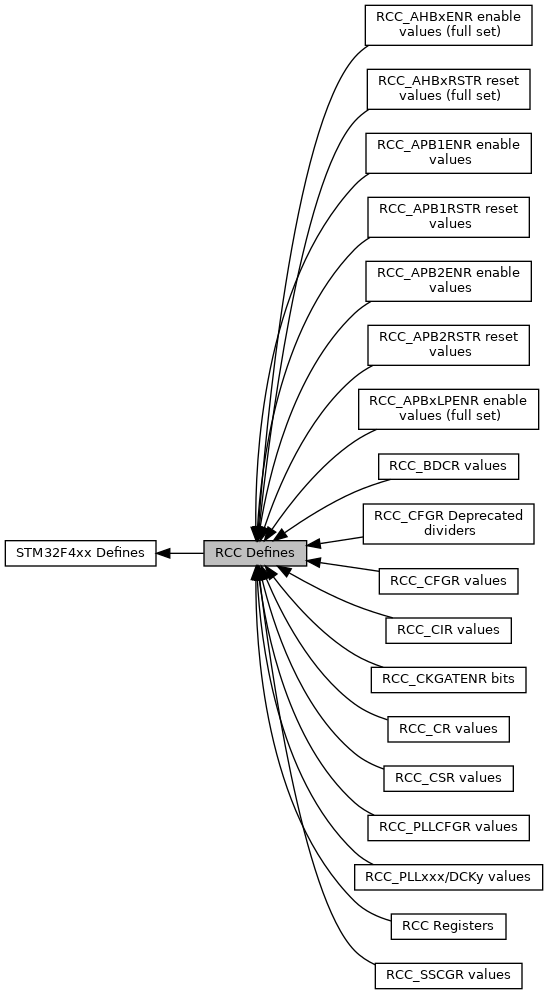## Data Structures

struct  rcc_clock_scale

## Modules

RCC Registers
Reset / Clock Control Registers.

RCC_CR values
Clock Control register values.

RCC_PLLCFGR values
PLL Configuration register values.

RCC_CFGR values
Clock Configuration register values.

RCC_CFGR Deprecated dividers
Older compatible definitions to ease migration.

RCC_CIR values
Clock Interrupt register values.

RCC_AHBxRSTR reset values (full set)

RCC_APB1RSTR reset values

RCC_APB2RSTR reset values

RCC_AHBxENR enable values (full set)

RCC_APB1ENR enable values

RCC_APB2ENR enable values

RCC_APBxLPENR enable values (full set)

RCC_BDCR values
Backup Domain control register values.

RCC_CSR values
Clock control and status register values.

RCC_SSCGR values
Spread spectrum clock generation register values.

RCC_PLLxxx/DCKy values
PLL and other dedicated clock register values.

RCC_CKGATENR bits
Allows to enable or disable the clock gating for the specified IPs.

## Macros

#define RCC_AHB1LPENR_OTGHSULPILPEN   (1 << 30)

#define RCC_AHB1LPENR_OTGHSLPEN   (1 << 29)

#define RCC_AHB1LPENR_ETHMACPTPLPEN   (1 << 28)

#define RCC_AHB1LPENR_ETHMACRXLPEN   (1 << 27)

#define RCC_AHB1LPENR_ETHMACTXLPEN   (1 << 26)

#define RCC_AHB1LPENR_ETHMACLPEN   (1 << 25)

#define RCC_AHB1LPENR_DMA2DLPEN   (1 << 23)

#define RCC_AHB1LPENR_DMA2LPEN   (1 << 22)

#define RCC_AHB1LPENR_DMA1LPEN   (1 << 21)

#define RCC_AHB1LPENR_SRAM3LPEN   (1 << 19)

#define RCC_AHB1LPENR_BKPSRAMLPEN   (1 << 18)

#define RCC_AHB1LPENR_SRAM2LPEN   (1 << 17)

#define RCC_AHB1LPENR_SRAM1LPEN   (1 << 16)

#define RCC_AHB1LPENR_FLITFLPEN   (1 << 15)

#define RCC_AHB1LPENR_CRCLPEN   (1 << 12)

#define RCC_AHB1LPENR_GPIOKLPEN   (1 << 10)

#define RCC_AHB1LPENR_GPIOJLPEN   (1 << 9)

#define RCC_AHB1LPENR_GPIOILPEN   (1 << 8)

#define RCC_AHB1LPENR_GPIOHLPEN   (1 << 7)

#define RCC_AHB1LPENR_GPIOGLPEN   (1 << 6)

#define RCC_AHB1LPENR_GPIOFLPEN   (1 << 5)

#define RCC_AHB1LPENR_GPIOELPEN   (1 << 4)

#define RCC_AHB1LPENR_GPIODLPEN   (1 << 3)

#define RCC_AHB1LPENR_GPIOCLPEN   (1 << 2)

#define RCC_AHB1LPENR_GPIOBLPEN   (1 << 1)

#define RCC_AHB1LPENR_GPIOALPEN   (1 << 0)

#define RCC_AHB2LPENR_OTGFSLPEN   (1 << 7)

#define RCC_AHB2LPENR_RNGLPEN   (1 << 6)

#define RCC_AHB2LPENR_HASHLPEN   (1 << 5)

#define RCC_AHB2LPENR_CRYPLPEN   (1 << 4)

#define RCC_AHB2LPENR_DCMILPEN   (1 << 0)

#define RCC_AHB3LPENR_QSPIEN   (1 << 1)

#define RCC_AHB3LPENR_FSMCLPEN   (1 << 0)

#define RCC_AHB3LPENR_FMCLPEN   (1 << 0)

#define _REG_BIT(base, bit)   (((base) << 5) + (bit))

## Enumerations

enum  rcc_clock_3v3 { RCC_CLOCK_3V3_84MHZ , RCC_CLOCK_3V3_168MHZ , RCC_CLOCK_3V3_180MHZ , RCC_CLOCK_3V3_END }

enum  rcc_osc {
RCC_PLL , RCC_PLLSAI , RCC_PLLI2S , RCC_HSE ,
RCC_HSI , RCC_LSE , RCC_LSI
}

enum  rcc_periph_clken {
RCC_GPIOA = _REG_BIT(0x30, 0) , RCC_GPIOB = _REG_BIT(0x30, 1) , RCC_GPIOC = _REG_BIT(0x30, 2) , RCC_GPIOD = _REG_BIT(0x30, 3) ,
RCC_GPIOE = _REG_BIT(0x30, 4) , RCC_GPIOF = _REG_BIT(0x30, 5) , RCC_GPIOG = _REG_BIT(0x30, 6) , RCC_GPIOH = _REG_BIT(0x30, 7) ,
RCC_GPIOI = _REG_BIT(0x30, 8) , RCC_GPIOJ = _REG_BIT(0x30, 9) , RCC_GPIOK = _REG_BIT(0x30, 10) , RCC_CRC = _REG_BIT(0x30, 12) ,
RCC_BKPSRAM = _REG_BIT(0x30, 18) , RCC_CCMDATARAM = _REG_BIT(0x30, 20) , RCC_DMA1 = _REG_BIT(0x30, 21) , RCC_DMA2 = _REG_BIT(0x30, 22) ,
RCC_DMA2D = _REG_BIT(0x30, 23) , RCC_ETHMAC = _REG_BIT(0x30, 25) , RCC_ETHMACTX = _REG_BIT(0x30, 26) , RCC_ETHMACRX = _REG_BIT(0x30, 27) ,
RCC_ETHMACPTP = _REG_BIT(0x30, 28) , RCC_OTGHS = _REG_BIT(0x30, 29) , RCC_OTGHSULPI = _REG_BIT(0x30, 30) , RCC_DCMI = _REG_BIT(0x34, 0) ,
RCC_CRYP = _REG_BIT(0x34, 4) , RCC_HASH = _REG_BIT(0x34, 5) , RCC_RNG = _REG_BIT(0x34, 6) , RCC_OTGFS = _REG_BIT(0x34, 7) ,
RCC_FSMC = _REG_BIT(0x38, 0) , RCC_FMC = _REG_BIT(0x38, 0) , RCC_QUADSPI = _REG_BIT(0x38, 1) , RCC_TIM2 = _REG_BIT(0x40, 0) ,
RCC_TIM3 = _REG_BIT(0x40, 1) , RCC_TIM4 = _REG_BIT(0x40, 2) , RCC_TIM5 = _REG_BIT(0x40, 3) , RCC_TIM6 = _REG_BIT(0x40, 4) ,
RCC_TIM7 = _REG_BIT(0x40, 5) , RCC_TIM12 = _REG_BIT(0x40, 6) , RCC_TIM13 = _REG_BIT(0x40, 7) , RCC_TIM14 = _REG_BIT(0x40, 8) ,
RCC_WWDG = _REG_BIT(0x40, 11) , RCC_SPI2 = _REG_BIT(0x40, 14) , RCC_SPI3 = _REG_BIT(0x40, 15) , RCC_USART2 = _REG_BIT(0x40, 17) ,
RCC_USART3 = _REG_BIT(0x40, 18) , RCC_UART4 = _REG_BIT(0x40, 19) , RCC_UART5 = _REG_BIT(0x40, 20) , RCC_I2C1 = _REG_BIT(0x40, 21) ,
RCC_I2C2 = _REG_BIT(0x40, 22) , RCC_I2C3 = _REG_BIT(0x40, 23) , RCC_CAN1 = _REG_BIT(0x40, 25) , RCC_CAN2 = _REG_BIT(0x40, 26) ,
RCC_PWR = _REG_BIT(0x40, 28) , RCC_DAC = _REG_BIT(0x40, 29) , RCC_UART7 = _REG_BIT(0x40, 30) , RCC_UART8 = _REG_BIT(0x40, 31) ,
RCC_TIM1 = _REG_BIT(0x44, 0) , RCC_TIM8 = _REG_BIT(0x44, 1) , RCC_USART1 = _REG_BIT(0x44, 4) , RCC_USART6 = _REG_BIT(0x44, 5) ,
RCC_ADC1 = _REG_BIT(0x44, 8) , RCC_ADC2 = _REG_BIT(0x44, 9) , RCC_ADC3 = _REG_BIT(0x44, 10) , RCC_SDIO = _REG_BIT(0x44, 11) ,
RCC_SPI1 = _REG_BIT(0x44, 12) , RCC_SPI4 = _REG_BIT(0x44, 13) , RCC_SYSCFG = _REG_BIT(0x44, 14) , RCC_TIM9 = _REG_BIT(0x44, 16) ,
RCC_TIM10 = _REG_BIT(0x44, 17) , RCC_TIM11 = _REG_BIT(0x44, 18) , RCC_SPI5 = _REG_BIT(0x44, 20) , RCC_SPI6 = _REG_BIT(0x44, 21) ,
RCC_SAI1EN = _REG_BIT(0x44, 22) , RCC_LTDC = _REG_BIT(0x44, 26) , RCC_DSI = _REG_BIT(0x44, 27) , RCC_RTC = _REG_BIT(0x70, 15) ,
SCC_GPIOA = _REG_BIT(0x50, 0) , SCC_GPIOB = _REG_BIT(0x50, 1) , SCC_GPIOC = _REG_BIT(0x50, 2) , SCC_GPIOD = _REG_BIT(0x50, 3) ,
SCC_GPIOE = _REG_BIT(0x50, 4) , SCC_GPIOF = _REG_BIT(0x50, 5) , SCC_GPIOG = _REG_BIT(0x50, 6) , SCC_GPIOH = _REG_BIT(0x50, 7) ,
SCC_GPIOI = _REG_BIT(0x50, 8) , SCC_GPIOJ = _REG_BIT(0x50, 9) , SCC_GPIOK = _REG_BIT(0x50, 10) , SCC_CRC = _REG_BIT(0x50, 12) ,
SCC_FLTIF = _REG_BIT(0x50, 15) , SCC_SRAM1 = _REG_BIT(0x50, 16) , SCC_SRAM2 = _REG_BIT(0x50, 17) , SCC_BKPSRAM = _REG_BIT(0x50, 18) ,
SCC_SRAM3 = _REG_BIT(0x50, 19) , SCC_DMA1 = _REG_BIT(0x50, 21) , SCC_DMA2 = _REG_BIT(0x50, 22) , SCC_DMA2D = _REG_BIT(0x50, 23) ,
SCC_ETHMAC = _REG_BIT(0x50, 25) , SCC_ETHMACTX = _REG_BIT(0x50, 26) , SCC_ETHMACRX = _REG_BIT(0x50, 27) , SCC_ETHMACPTP = _REG_BIT(0x50, 28) ,
SCC_OTGHS = _REG_BIT(0x50, 29) , SCC_OTGHSULPI = _REG_BIT(0x50, 30) , SCC_DCMI = _REG_BIT(0x54, 0) , SCC_CRYP = _REG_BIT(0x54, 4) ,
SCC_HASH = _REG_BIT(0x54, 5) , SCC_RNG = _REG_BIT(0x54, 6) , SCC_OTGFS = _REG_BIT(0x54, 7) , SCC_QSPIC = _REG_BIT(0x58, 1) ,
SCC_FMC = _REG_BIT(0x58, 0) , SCC_FSMC = _REG_BIT(0x58, 0) , SCC_TIM2 = _REG_BIT(0x60, 0) , SCC_TIM3 = _REG_BIT(0x60, 1) ,
SCC_TIM4 = _REG_BIT(0x60, 2) , SCC_TIM5 = _REG_BIT(0x60, 3) , SCC_TIM6 = _REG_BIT(0x60, 4) , SCC_TIM7 = _REG_BIT(0x60, 5) ,
SCC_TIM12 = _REG_BIT(0x60, 6) , SCC_TIM13 = _REG_BIT(0x60, 7) , SCC_TIM14 = _REG_BIT(0x60, 8) , SCC_WWDG = _REG_BIT(0x60, 11) ,
SCC_SPI2 = _REG_BIT(0x60, 14) , SCC_SPI3 = _REG_BIT(0x60, 15) , SCC_USART2 = _REG_BIT(0x60, 17) , SCC_USART3 = _REG_BIT(0x60, 18) ,
SCC_UART4 = _REG_BIT(0x60, 19) , SCC_UART5 = _REG_BIT(0x60, 20) , SCC_I2C1 = _REG_BIT(0x60, 21) , SCC_I2C2 = _REG_BIT(0x60, 22) ,
SCC_I2C3 = _REG_BIT(0x60, 23) , SCC_CAN1 = _REG_BIT(0x60, 25) , SCC_CAN2 = _REG_BIT(0x60, 26) , SCC_PWR = _REG_BIT(0x60, 28) ,
SCC_DAC = _REG_BIT(0x60, 29) , SCC_UART7 = _REG_BIT(0x60, 30) , SCC_UART8 = _REG_BIT(0x60, 31) , SCC_TIM1 = _REG_BIT(0x64, 0) ,
SCC_TIM8 = _REG_BIT(0x64, 1) , SCC_USART1 = _REG_BIT(0x64, 4) , SCC_USART6 = _REG_BIT(0x64, 5) , SCC_ADC1 = _REG_BIT(0x64, 8) ,
SCC_ADC2 = _REG_BIT(0x64, 9) , SCC_ADC3 = _REG_BIT(0x64, 10) , SCC_SDIO = _REG_BIT(0x64, 11) , SCC_SPI1 = _REG_BIT(0x64, 12) ,
SCC_SPI4 = _REG_BIT(0x64, 13) , SCC_SYSCFG = _REG_BIT(0x64, 14) , SCC_TIM9 = _REG_BIT(0x64, 16) , SCC_TIM10 = _REG_BIT(0x64, 17) ,
SCC_TIM11 = _REG_BIT(0x64, 18) , SCC_SPI5 = _REG_BIT(0x64, 20) , SCC_SPI6 = _REG_BIT(0x64, 21) , SCC_SAI1 = _REG_BIT(0x64, 22) ,
SCC_LTDC = _REG_BIT(0x64, 26) , SCC_DSI = _REG_BIT(0x64, 27)
}

enum  rcc_periph_rst {
RST_GPIOA = _REG_BIT(0x10, 0) , RST_GPIOB = _REG_BIT(0x10, 1) , RST_GPIOC = _REG_BIT(0x10, 2) , RST_GPIOD = _REG_BIT(0x10, 3) ,
RST_GPIOE = _REG_BIT(0x10, 4) , RST_GPIOF = _REG_BIT(0x10, 5) , RST_GPIOG = _REG_BIT(0x10, 6) , RST_GPIOH = _REG_BIT(0x10, 7) ,
RST_GPIOI = _REG_BIT(0x10, 8) , RST_GPIOJ = _REG_BIT(0x10, 9) , RST_GPIOK = _REG_BIT(0x10, 10) , RST_CRC = _REG_BIT(0x10, 12) ,
RST_DMA1 = _REG_BIT(0x10, 21) , RST_DMA2 = _REG_BIT(0x10, 22) , RST_DMA2D = _REG_BIT(0x10, 23) , RST_ETHMAC = _REG_BIT(0x10, 25) ,
RST_OTGHS = _REG_BIT(0x10, 29) , RST_DCMI = _REG_BIT(0x14, 0) , RST_CRYP = _REG_BIT(0x14, 4) , RST_HASH = _REG_BIT(0x14, 5) ,
RST_RNG = _REG_BIT(0x14, 6) , RST_OTGFS = _REG_BIT(0x14, 7) , RST_QSPI = _REG_BIT(0x18, 1) , RST_FSMC = _REG_BIT(0x18, 0) ,
RST_FMC = _REG_BIT(0x18, 0) , RST_TIM2 = _REG_BIT(0x20, 0) , RST_TIM3 = _REG_BIT(0x20, 1) , RST_TIM4 = _REG_BIT(0x20, 2) ,
RST_TIM5 = _REG_BIT(0x20, 3) , RST_TIM6 = _REG_BIT(0x20, 4) , RST_TIM7 = _REG_BIT(0x20, 5) , RST_TIM12 = _REG_BIT(0x20, 6) ,
RST_TIM13 = _REG_BIT(0x20, 7) , RST_TIM14 = _REG_BIT(0x20, 8) , RST_WWDG = _REG_BIT(0x20, 11) , RST_SPI2 = _REG_BIT(0x20, 14) ,
RST_SPI3 = _REG_BIT(0x20, 15) , RST_USART2 = _REG_BIT(0x20, 17) , RST_USART3 = _REG_BIT(0x20, 18) , RST_UART4 = _REG_BIT(0x20, 19) ,
RST_UART5 = _REG_BIT(0x20, 20) , RST_I2C1 = _REG_BIT(0x20, 21) , RST_I2C2 = _REG_BIT(0x20, 22) , RST_I2C3 = _REG_BIT(0x20, 23) ,
RST_CAN1 = _REG_BIT(0x20, 25) , RST_CAN2 = _REG_BIT(0x20, 26) , RST_PWR = _REG_BIT(0x20, 28) , RST_DAC = _REG_BIT(0x20, 29) ,
RST_UART7 = _REG_BIT(0x20, 30) , RST_UART8 = _REG_BIT(0x20, 31) , RST_TIM1 = _REG_BIT(0x24, 0) , RST_TIM8 = _REG_BIT(0x24, 1) ,
RST_USART1 = _REG_BIT(0x24, 4) , RST_USART6 = _REG_BIT(0x24, 5) , RST_ADC = _REG_BIT(0x24, 8) , RST_SDIO = _REG_BIT(0x24, 11) ,
RST_SPI1 = _REG_BIT(0x24, 12) , RST_SPI4 = _REG_BIT(0x24, 13) , RST_SYSCFG = _REG_BIT(0x24, 14) , RST_TIM9 = _REG_BIT(0x24, 16) ,
RST_TIM10 = _REG_BIT(0x24, 17) , RST_TIM11 = _REG_BIT(0x24, 18) , RST_SPI5 = _REG_BIT(0x24, 20) , RST_SPI6 = _REG_BIT(0x24, 21) ,
RST_SAI1RST = _REG_BIT(0x24, 22) , RST_LTDC = _REG_BIT(0x24, 26) , RST_DSI = _REG_BIT(0x24, 27) , RST_BDCR = _REG_BIT(0x70, 16)
}

## Functions

void rcc_osc_ready_int_clear (enum rcc_osc osc)

void rcc_osc_ready_int_enable (enum rcc_osc osc)

void rcc_osc_ready_int_disable (enum rcc_osc osc)

int rcc_osc_ready_int_flag (enum rcc_osc osc)

void rcc_css_int_clear (void)

int rcc_css_int_flag (void)

void rcc_wait_for_sysclk_status (enum rcc_osc osc)

void rcc_osc_on (enum rcc_osc osc)

void rcc_osc_off (enum rcc_osc osc)

void rcc_css_enable (void)

void rcc_css_disable (void)

void rcc_plli2s_config (uint16_t n, uint8_t r)
Set the dividers for the PLLI2S clock outputs. More...

void rcc_pllsai_config (uint16_t n, uint16_t p, uint16_t q, uint16_t r)
Set the dividers for the PLLSAI clock outputs divider p is only available on F4x9 parts, pass 0 for other parts. More...

void rcc_pllsai_postscalers (uint8_t q, uint8_t r)
Set the dedicated dividers after the PLLSAI configuration. More...

void rcc_set_sysclk_source (uint32_t clk)

void rcc_set_pll_source (uint32_t pllsrc)

void rcc_set_ppre2 (uint32_t ppre2)

void rcc_set_ppre1 (uint32_t ppre1)

void rcc_set_hpre (uint32_t hpre)

void rcc_set_rtcpre (uint32_t rtcpre)

void rcc_set_main_pll_hsi (uint32_t pllm, uint32_t plln, uint32_t pllp, uint32_t pllq, uint32_t pllr)
Reconfigures the main PLL for a HSI source. More...

void rcc_set_main_pll_hse (uint32_t pllm, uint32_t plln, uint32_t pllp, uint32_t pllq, uint32_t pllr)
Reconfigures the main PLL for a HSE source. More...

uint32_t rcc_system_clock_source (void)

void rcc_clock_setup_pll (const struct rcc_clock_scale *clock)
Setup clocks to run from PLL. More...

void rcc_clock_setup_hse_3v3 (const struct rcc_clock_scale *clock)
Setup clocks with the HSE. More...

uint32_t rcc_get_usart_clk_freq (uint32_t usart)
Get the peripheral clock speed for the USART at base specified. More...

uint32_t rcc_get_timer_clk_freq (uint32_t timer)
Get the peripheral clock speed for the Timer at base specified. More...

uint32_t rcc_get_i2c_clk_freq (uint32_t i2c)
Get the peripheral clock speed for the I2C device at base specified. More...

uint32_t rcc_get_spi_clk_freq (uint32_t spi)
Get the peripheral clock speed for the SPI device at base specified. More...

void rcc_peripheral_enable_clock (volatile uint32_t *reg, uint32_t en)
RCC Enable Peripheral Clocks. More...

void rcc_peripheral_disable_clock (volatile uint32_t *reg, uint32_t en)
RCC Disable Peripheral Clocks. More...

void rcc_peripheral_reset (volatile uint32_t *reg, uint32_t reset)
RCC Reset Peripherals. More...

void rcc_peripheral_clear_reset (volatile uint32_t *reg, uint32_t clear_reset)
RCC Remove Reset on Peripherals. More...

void rcc_periph_clock_enable (enum rcc_periph_clken clken)
Enable Peripheral Clock in running mode. More...

void rcc_periph_clock_disable (enum rcc_periph_clken clken)
Disable Peripheral Clock in running mode. More...

void rcc_periph_reset_pulse (enum rcc_periph_rst rst)
Reset Peripheral, pulsed. More...

void rcc_periph_reset_hold (enum rcc_periph_rst rst)
Reset Peripheral, hold. More...

void rcc_periph_reset_release (enum rcc_periph_rst rst)
Reset Peripheral, release. More...

void rcc_set_mco (uint32_t mcosrc)
Select the source of Microcontroller Clock Output. More...

void rcc_osc_bypass_enable (enum rcc_osc osc)
RCC Enable Bypass. More...

void rcc_osc_bypass_disable (enum rcc_osc osc)
RCC Disable Bypass. More...

bool rcc_is_osc_ready (enum rcc_osc osc)
Is the given oscillator ready? More...

void rcc_wait_for_osc_ready (enum rcc_osc osc)
Wait for Oscillator Ready. More...

uint16_t rcc_get_div_from_hpre (uint8_t div_val)
This will return the divisor 1/2/4/8/16/64/128/256/512 which is set as a 4-bit value, typically used for hpre and other prescalers. More...

## Variables

uint32_t rcc_ahb_frequency

uint32_t rcc_apb1_frequency

uint32_t rcc_apb2_frequency

const struct rcc_clock_scale rcc_hsi_configs [RCC_CLOCK_3V3_END]

const struct rcc_clock_scale rcc_hse_8mhz_3v3 [RCC_CLOCK_3V3_END]

const struct rcc_clock_scale rcc_hse_12mhz_3v3 [RCC_CLOCK_3V3_END]

const struct rcc_clock_scale rcc_hse_16mhz_3v3 [RCC_CLOCK_3V3_END]

const struct rcc_clock_scale rcc_hse_25mhz_3v3 [RCC_CLOCK_3V3_END]

## Detailed Description

Defined Constants and Types for the STM32F4xx Reset and Clock Control

Version
1.0.0
Date
18 August 2012

LGPL License Terms libopencm3 License

## ◆ _REG_BIT

 #define _REG_BIT ( base, bit ) (((base) << 5) + (bit))

Definition at line 837 of file f4/rcc.h.

## ◆ RCC_AHB1LPENR_BKPSRAMLPEN

 #define RCC_AHB1LPENR_BKPSRAMLPEN   (1 << 18)

Definition at line 547 of file f4/rcc.h.

## ◆ RCC_AHB1LPENR_CRCLPEN

 #define RCC_AHB1LPENR_CRCLPEN   (1 << 12)

Definition at line 551 of file f4/rcc.h.

## ◆ RCC_AHB1LPENR_DMA1LPEN

 #define RCC_AHB1LPENR_DMA1LPEN   (1 << 21)

Definition at line 545 of file f4/rcc.h.

## ◆ RCC_AHB1LPENR_DMA2DLPEN

 #define RCC_AHB1LPENR_DMA2DLPEN   (1 << 23)

Definition at line 543 of file f4/rcc.h.

## ◆ RCC_AHB1LPENR_DMA2LPEN

 #define RCC_AHB1LPENR_DMA2LPEN   (1 << 22)

Definition at line 544 of file f4/rcc.h.

## ◆ RCC_AHB1LPENR_ETHMACLPEN

 #define RCC_AHB1LPENR_ETHMACLPEN   (1 << 25)

Definition at line 542 of file f4/rcc.h.

## ◆ RCC_AHB1LPENR_ETHMACPTPLPEN

 #define RCC_AHB1LPENR_ETHMACPTPLPEN   (1 << 28)

Definition at line 539 of file f4/rcc.h.

## ◆ RCC_AHB1LPENR_ETHMACRXLPEN

 #define RCC_AHB1LPENR_ETHMACRXLPEN   (1 << 27)

Definition at line 540 of file f4/rcc.h.

## ◆ RCC_AHB1LPENR_ETHMACTXLPEN

 #define RCC_AHB1LPENR_ETHMACTXLPEN   (1 << 26)

Definition at line 541 of file f4/rcc.h.

## ◆ RCC_AHB1LPENR_FLITFLPEN

 #define RCC_AHB1LPENR_FLITFLPEN   (1 << 15)

Definition at line 550 of file f4/rcc.h.

## ◆ RCC_AHB1LPENR_GPIOALPEN

 #define RCC_AHB1LPENR_GPIOALPEN   (1 << 0)

Definition at line 562 of file f4/rcc.h.

## ◆ RCC_AHB1LPENR_GPIOBLPEN

 #define RCC_AHB1LPENR_GPIOBLPEN   (1 << 1)

Definition at line 561 of file f4/rcc.h.

## ◆ RCC_AHB1LPENR_GPIOCLPEN

 #define RCC_AHB1LPENR_GPIOCLPEN   (1 << 2)

Definition at line 560 of file f4/rcc.h.

## ◆ RCC_AHB1LPENR_GPIODLPEN

 #define RCC_AHB1LPENR_GPIODLPEN   (1 << 3)

Definition at line 559 of file f4/rcc.h.

## ◆ RCC_AHB1LPENR_GPIOELPEN

 #define RCC_AHB1LPENR_GPIOELPEN   (1 << 4)

Definition at line 558 of file f4/rcc.h.

## ◆ RCC_AHB1LPENR_GPIOFLPEN

 #define RCC_AHB1LPENR_GPIOFLPEN   (1 << 5)

Definition at line 557 of file f4/rcc.h.

## ◆ RCC_AHB1LPENR_GPIOGLPEN

 #define RCC_AHB1LPENR_GPIOGLPEN   (1 << 6)

Definition at line 556 of file f4/rcc.h.

## ◆ RCC_AHB1LPENR_GPIOHLPEN

 #define RCC_AHB1LPENR_GPIOHLPEN   (1 << 7)

Definition at line 555 of file f4/rcc.h.

## ◆ RCC_AHB1LPENR_GPIOILPEN

 #define RCC_AHB1LPENR_GPIOILPEN   (1 << 8)

Definition at line 554 of file f4/rcc.h.

## ◆ RCC_AHB1LPENR_GPIOJLPEN

 #define RCC_AHB1LPENR_GPIOJLPEN   (1 << 9)

Definition at line 553 of file f4/rcc.h.

## ◆ RCC_AHB1LPENR_GPIOKLPEN

 #define RCC_AHB1LPENR_GPIOKLPEN   (1 << 10)

Definition at line 552 of file f4/rcc.h.

## ◆ RCC_AHB1LPENR_OTGHSLPEN

 #define RCC_AHB1LPENR_OTGHSLPEN   (1 << 29)

Definition at line 538 of file f4/rcc.h.

## ◆ RCC_AHB1LPENR_OTGHSULPILPEN

 #define RCC_AHB1LPENR_OTGHSULPILPEN   (1 << 30)

Definition at line 537 of file f4/rcc.h.

## ◆ RCC_AHB1LPENR_SRAM1LPEN

 #define RCC_AHB1LPENR_SRAM1LPEN   (1 << 16)

Definition at line 549 of file f4/rcc.h.

## ◆ RCC_AHB1LPENR_SRAM2LPEN

 #define RCC_AHB1LPENR_SRAM2LPEN   (1 << 17)

Definition at line 548 of file f4/rcc.h.

## ◆ RCC_AHB1LPENR_SRAM3LPEN

 #define RCC_AHB1LPENR_SRAM3LPEN   (1 << 19)

Definition at line 546 of file f4/rcc.h.

## ◆ RCC_AHB2LPENR_CRYPLPEN

 #define RCC_AHB2LPENR_CRYPLPEN   (1 << 4)

Definition at line 586 of file f4/rcc.h.

## ◆ RCC_AHB2LPENR_DCMILPEN

 #define RCC_AHB2LPENR_DCMILPEN   (1 << 0)

Definition at line 587 of file f4/rcc.h.

## ◆ RCC_AHB2LPENR_HASHLPEN

 #define RCC_AHB2LPENR_HASHLPEN   (1 << 5)

Definition at line 585 of file f4/rcc.h.

## ◆ RCC_AHB2LPENR_OTGFSLPEN

 #define RCC_AHB2LPENR_OTGFSLPEN   (1 << 7)

Definition at line 583 of file f4/rcc.h.

## ◆ RCC_AHB2LPENR_RNGLPEN

 #define RCC_AHB2LPENR_RNGLPEN   (1 << 6)

Definition at line 584 of file f4/rcc.h.

## ◆ RCC_AHB3LPENR_FMCLPEN

 #define RCC_AHB3LPENR_FMCLPEN   (1 << 0)

Definition at line 593 of file f4/rcc.h.

## ◆ RCC_AHB3LPENR_FSMCLPEN

 #define RCC_AHB3LPENR_FSMCLPEN   (1 << 0)

Definition at line 592 of file f4/rcc.h.

## ◆ RCC_AHB3LPENR_QSPIEN

 #define RCC_AHB3LPENR_QSPIEN   (1 << 1)

Definition at line 591 of file f4/rcc.h.

## ◆ rcc_clock_3v3

 enum rcc_clock_3v3
Enumerator
RCC_CLOCK_3V3_84MHZ
RCC_CLOCK_3V3_168MHZ
RCC_CLOCK_3V3_180MHZ
RCC_CLOCK_3V3_END

Definition at line 797 of file f4/rcc.h.

## ◆ rcc_osc

 enum rcc_osc
Enumerator
RCC_PLL
RCC_PLLSAI
RCC_PLLI2S
RCC_HSE
RCC_HSI
RCC_LSE
RCC_LSI

Definition at line 827 of file f4/rcc.h.

## ◆ rcc_periph_clken

 enum rcc_periph_clken
Enumerator
RCC_GPIOA
RCC_GPIOB
RCC_GPIOC
RCC_GPIOD
RCC_GPIOE
RCC_GPIOF
RCC_GPIOG
RCC_GPIOH
RCC_GPIOI
RCC_GPIOJ
RCC_GPIOK
RCC_CRC
RCC_BKPSRAM
RCC_CCMDATARAM
RCC_DMA1
RCC_DMA2
RCC_DMA2D
RCC_ETHMAC
RCC_ETHMACTX
RCC_ETHMACRX
RCC_ETHMACPTP
RCC_OTGHS
RCC_OTGHSULPI
RCC_DCMI
RCC_CRYP
RCC_HASH
RCC_RNG
RCC_OTGFS
RCC_FSMC
RCC_FMC
RCC_QUADSPI
RCC_TIM2
RCC_TIM3
RCC_TIM4
RCC_TIM5
RCC_TIM6
RCC_TIM7
RCC_TIM12
RCC_TIM13
RCC_TIM14
RCC_WWDG
RCC_SPI2
RCC_SPI3
RCC_USART2
RCC_USART3
RCC_UART4
RCC_UART5
RCC_I2C1
RCC_I2C2
RCC_I2C3
RCC_CAN1
RCC_CAN2
RCC_PWR
RCC_DAC
RCC_UART7
RCC_UART8
RCC_TIM1
RCC_TIM8
RCC_USART1
RCC_USART6
RCC_ADC1
RCC_ADC2
RCC_ADC3
RCC_SDIO
RCC_SPI1
RCC_SPI4
RCC_SYSCFG
RCC_TIM9
RCC_TIM10
RCC_TIM11
RCC_SPI5
RCC_SPI6
RCC_SAI1EN
RCC_LTDC
RCC_DSI
RCC_RTC
SCC_GPIOA
SCC_GPIOB
SCC_GPIOC
SCC_GPIOD
SCC_GPIOE
SCC_GPIOF
SCC_GPIOG
SCC_GPIOH
SCC_GPIOI
SCC_GPIOJ
SCC_GPIOK
SCC_CRC
SCC_FLTIF
SCC_SRAM1
SCC_SRAM2
SCC_BKPSRAM
SCC_SRAM3
SCC_DMA1
SCC_DMA2
SCC_DMA2D
SCC_ETHMAC
SCC_ETHMACTX
SCC_ETHMACRX
SCC_ETHMACPTP
SCC_OTGHS
SCC_OTGHSULPI
SCC_DCMI
SCC_CRYP
SCC_HASH
SCC_RNG
SCC_OTGFS
SCC_QSPIC
SCC_FMC
SCC_FSMC
SCC_TIM2
SCC_TIM3
SCC_TIM4
SCC_TIM5
SCC_TIM6
SCC_TIM7
SCC_TIM12
SCC_TIM13
SCC_TIM14
SCC_WWDG
SCC_SPI2
SCC_SPI3
SCC_USART2
SCC_USART3
SCC_UART4
SCC_UART5
SCC_I2C1
SCC_I2C2
SCC_I2C3
SCC_CAN1
SCC_CAN2
SCC_PWR
SCC_DAC
SCC_UART7
SCC_UART8
SCC_TIM1
SCC_TIM8
SCC_USART1
SCC_USART6
SCC_ADC1
SCC_ADC2
SCC_ADC3
SCC_SDIO
SCC_SPI1
SCC_SPI4
SCC_SYSCFG
SCC_TIM9
SCC_TIM10
SCC_TIM11
SCC_SPI5
SCC_SPI6
SCC_SAI1
SCC_LTDC
SCC_DSI

Definition at line 839 of file f4/rcc.h.

## ◆ rcc_periph_rst

 enum rcc_periph_rst
Enumerator
RST_GPIOA
RST_GPIOB
RST_GPIOC
RST_GPIOD
RST_GPIOE
RST_GPIOF
RST_GPIOG
RST_GPIOH
RST_GPIOI
RST_GPIOJ
RST_GPIOK
RST_CRC
RST_DMA1
RST_DMA2
RST_DMA2D
RST_ETHMAC
RST_OTGHS
RST_DCMI
RST_CRYP
RST_HASH
RST_RNG
RST_OTGFS
RST_QSPI
RST_FSMC
RST_FMC
RST_TIM2
RST_TIM3
RST_TIM4
RST_TIM5
RST_TIM6
RST_TIM7
RST_TIM12
RST_TIM13
RST_TIM14
RST_WWDG
RST_SPI2
RST_SPI3
RST_USART2
RST_USART3
RST_UART4
RST_UART5
RST_I2C1
RST_I2C2
RST_I2C3
RST_CAN1
RST_CAN2
RST_PWR
RST_DAC
RST_UART7
RST_UART8
RST_TIM1
RST_TIM8
RST_USART1
RST_USART6
RST_ADC
RST_SDIO
RST_SPI1
RST_SPI4
RST_SYSCFG
RST_TIM9
RST_TIM10
RST_TIM11
RST_SPI5
RST_SPI6
RST_SAI1RST
RST_LTDC
RST_DSI
RST_BDCR

Definition at line 1018 of file f4/rcc.h.

## ◆ rcc_clock_setup_hse_3v3()

 void rcc_clock_setup_hse_3v3 ( const struct rcc_clock_scale * clock )

Setup clocks with the HSE.

Deprecated:
replaced by rcc_clock_setup_pll as a drop in replacement.
See also
rcc_clock_setup_pll which supports HSI as well as HSE, using the same clock structures.

Definition at line 789 of file rcc.c.

References rcc_clock_setup_pll().

Here is the call graph for this function: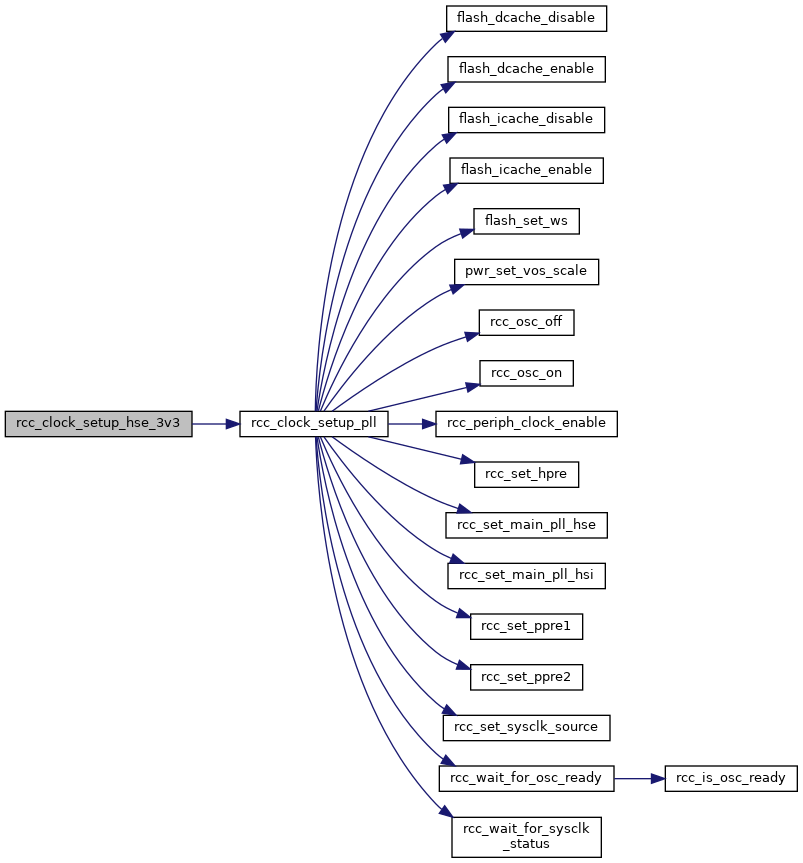## ◆ rcc_clock_setup_pll()

 void rcc_clock_setup_pll ( const struct rcc_clock_scale * clock )

Setup clocks to run from PLL.

The arguments provide the pll source, multipliers, dividers, all that's needed to establish a system clock.

Parameters
 clock clock information structure.

Definition at line 709 of file rcc.c.

Referenced by rcc_clock_setup_hse_3v3().

Here is the call graph for this function: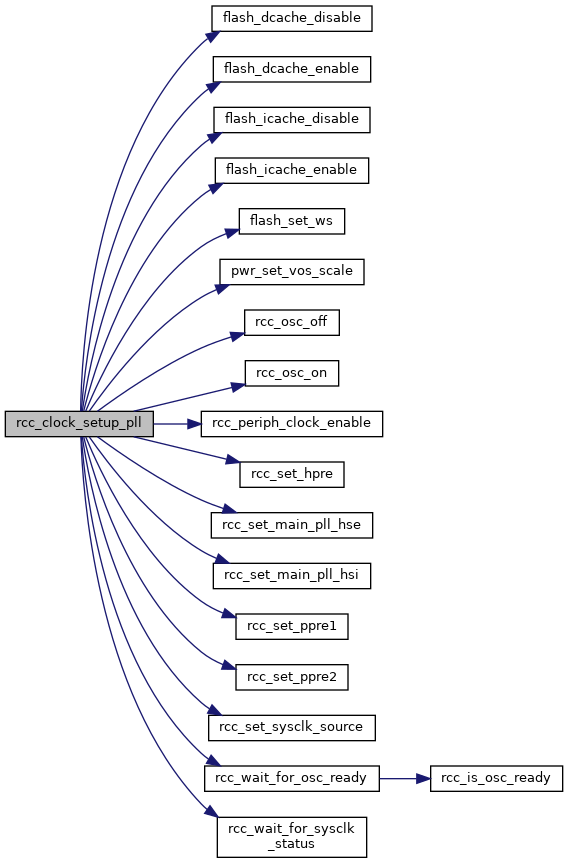Here is the caller graph for this function: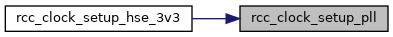## ◆ rcc_css_disable()

 void rcc_css_disable ( void )

Definition at line 540 of file rcc.c.

References RCC_CR, and RCC_CR_CSSON.

## ◆ rcc_css_enable()

 void rcc_css_enable ( void )

Definition at line 535 of file rcc.c.

References RCC_CR, and RCC_CR_CSSON.

## ◆ rcc_css_int_clear()

 void rcc_css_int_clear ( void )

Definition at line 424 of file rcc.c.

References RCC_CIR, and RCC_CIR_CSSC.

## ◆ rcc_css_int_flag()

 int rcc_css_int_flag ( void )

Definition at line 429 of file rcc.c.

References RCC_CIR, and RCC_CIR_CSSF.

## ◆ rcc_get_div_from_hpre()

 uint16_t rcc_get_div_from_hpre ( uint8_t div_val )

This will return the divisor 1/2/4/8/16/64/128/256/512 which is set as a 4-bit value, typically used for hpre and other prescalers.

Parameters
 div_val Masked and shifted divider value from register (e.g. RCC_CFGR)

Definition at line 276 of file rcc_common_all.c.

## ◆ rcc_get_i2c_clk_freq()

 uint32_t rcc_get_i2c_clk_freq ( uint32_t i2c )

Get the peripheral clock speed for the I2C device at base specified.

Parameters
 i2c Base address of I2C to get clock frequency for.

Definition at line 830 of file rcc.c.

References rcc_apb1_frequency.

## ◆ rcc_get_spi_clk_freq()

 uint32_t rcc_get_spi_clk_freq ( uint32_t spi )

Get the peripheral clock speed for the SPI device at base specified.

Parameters
 spi Base address of SPI device to get clock frequency for (e.g. SPI1_BASE).

Definition at line 839 of file rcc.c.

References rcc_apb1_frequency, rcc_apb2_frequency, SPI2_BASE, and SPI3_BASE.

## ◆ rcc_get_timer_clk_freq()

 uint32_t rcc_get_timer_clk_freq ( uint32_t timer )

Get the peripheral clock speed for the Timer at base specified.

Parameters
 timer Base address of TIM to get clock frequency for.

Definition at line 812 of file rcc.c.

## ◆ rcc_get_usart_clk_freq()

 uint32_t rcc_get_usart_clk_freq ( uint32_t usart )

Get the peripheral clock speed for the USART at base specified.

Parameters
 usart Base address of USART to get clock frequency for.

Definition at line 798 of file rcc.c.

References rcc_apb1_frequency, rcc_apb2_frequency, USART1_BASE, and USART6_BASE.

## ◆ rcc_is_osc_ready()

 bool rcc_is_osc_ready ( enum rcc_osc osc )

Is the given oscillator ready?

Parameters
 osc Oscillator ID
Returns
true if the hardware indicates the oscillator is ready.

Definition at line 434 of file rcc.c.

Referenced by rcc_wait_for_osc_ready().

Here is the caller graph for this function: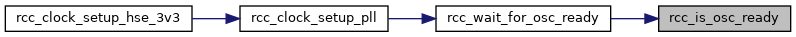## ◆ rcc_osc_bypass_disable()

 void rcc_osc_bypass_disable ( enum rcc_osc osc )

RCC Disable Bypass.

Re-enable the internal clock (high speed and low speed clocks only). The internal clock must be disabled (see rcc_osc_off) for this to have effect.

Note
The LSE clock is in the backup domain and cannot have bypass removed until the backup domain write protection has been removed (see pwr_disable_backup_domain_write_protect) or the backup domain has been reset (see rcc_backupdomain_reset).
Parameters
 [in] osc Oscillator ID. Only HSE and LSE have effect.

Definition at line 254 of file rcc_common_all.c.

References RCC_BDCR, RCC_BDCR_LSEBYP, RCC_CR, RCC_CR_HSEBYP, RCC_CSR, RCC_HSE, and RCC_LSE.

## ◆ rcc_osc_bypass_enable()

 void rcc_osc_bypass_enable ( enum rcc_osc osc )

RCC Enable Bypass.

Enable an external clock to bypass the internal clock (high speed and low speed clocks only). The external clock must be enabled (see rcc_osc_on) and the internal clock must be disabled (see rcc_osc_off) for this to have effect.

Note
The LSE clock is in the backup domain and cannot be bypassed until the backup domain write protection has been removed (see pwr_disable_backup_domain_write_protect).
Parameters
 [in] osc Oscillator ID. Only HSE and LSE have effect.

Definition at line 224 of file rcc_common_all.c.

References RCC_BDCR, RCC_BDCR_LSEBYP, RCC_CR, RCC_CR_HSEBYP, RCC_CSR, RCC_HSE, and RCC_LSE.

## ◆ rcc_osc_off()

 void rcc_osc_off ( enum rcc_osc osc )

Definition at line 508 of file rcc.c.

Referenced by rcc_clock_setup_pll().

Here is the caller graph for this function: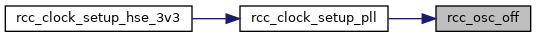## ◆ rcc_osc_on()

 void rcc_osc_on ( enum rcc_osc osc )

Definition at line 481 of file rcc.c.

Referenced by rcc_clock_setup_pll().

Here is the caller graph for this function: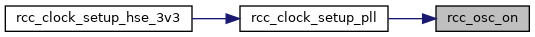## ◆ rcc_osc_ready_int_clear()

 void rcc_osc_ready_int_clear ( enum rcc_osc osc )

Definition at line 322 of file rcc.c.

## ◆ rcc_osc_ready_int_disable()

 void rcc_osc_ready_int_disable ( enum rcc_osc osc )

Definition at line 376 of file rcc.c.

## ◆ rcc_osc_ready_int_enable()

 void rcc_osc_ready_int_enable ( enum rcc_osc osc )

Definition at line 349 of file rcc.c.

## ◆ rcc_osc_ready_int_flag()

 int rcc_osc_ready_int_flag ( enum rcc_osc osc )

Definition at line 403 of file rcc.c.

## ◆ rcc_periph_clock_disable()

 void rcc_periph_clock_disable ( enum rcc_periph_clken clken )

Disable Peripheral Clock in running mode.

Disable the clock on particular peripheral.

Parameters
 [in] clken rcc_periph_clken Peripheral RCC

For available constants, see rcc_periph_clken (RCC_UART1 for example)

Definition at line 148 of file rcc_common_all.c.

References _RCC_BIT, and _RCC_REG.

## ◆ rcc_periph_clock_enable()

 void rcc_periph_clock_enable ( enum rcc_periph_clken clken )

Enable Peripheral Clock in running mode.

Enable the clock on particular peripheral.

Parameters
 [in] clken rcc_periph_clken Peripheral RCC

For available constants, see rcc_periph_clken (RCC_UART1 for example)

Definition at line 134 of file rcc_common_all.c.

References _RCC_BIT, and _RCC_REG.

Referenced by rcc_clock_setup_pll(), stm32f107_usbd_init(), and stm32f207_usbd_init().

Here is the caller graph for this function: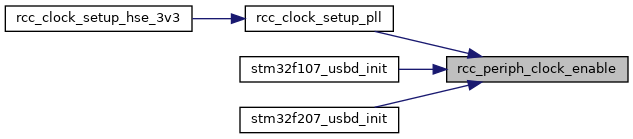## ◆ rcc_periph_reset_hold()

 void rcc_periph_reset_hold ( enum rcc_periph_rst rst )

Reset Peripheral, hold.

Reset particular peripheral, and hold in reset state.

Parameters
 [in] rst rcc_periph_rst Peripheral reset

For available constants, see rcc_periph_rst (RST_UART1 for example)

Definition at line 179 of file rcc_common_all.c.

References _RCC_BIT, and _RCC_REG.

## ◆ rcc_periph_reset_pulse()

 void rcc_periph_reset_pulse ( enum rcc_periph_rst rst )

Reset Peripheral, pulsed.

Reset particular peripheral, and restore to working state.

Parameters
 [in] rst rcc_periph_rst Peripheral reset

For available constants, see rcc_periph_rst (RST_UART1 for example)

Definition at line 163 of file rcc_common_all.c.

References _RCC_BIT, and _RCC_REG.

Referenced by can_reset(), i2c_reset(), and spi_reset().

Here is the caller graph for this function: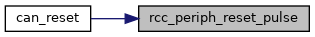## ◆ rcc_periph_reset_release()

 void rcc_periph_reset_release ( enum rcc_periph_rst rst )

Reset Peripheral, release.

Restore peripheral from reset state to working state.

Parameters
 [in] rst rcc_periph_rst Peripheral reset

For available constants, see rcc_periph_rst (RST_UART1 for example)

Definition at line 194 of file rcc_common_all.c.

References _RCC_BIT, and _RCC_REG.

## ◆ rcc_peripheral_clear_reset()

 void rcc_peripheral_clear_reset ( volatile uint32_t * reg, uint32_t clear_reset )

RCC Remove Reset on Peripherals.

Remove the reset on particular peripherals. There are three registers involved, each one controlling reset of peripherals associated with the AHB, APB1 and APB2 respectively. Several peripherals could have the reset removed simultaneously only if they are controlled by the same register.

See also
rcc_periph_reset_release for a less error prone version, if you only need to unreset a single peripheral.
rcc_periph_reset_pulse if you are only going to toggle reset anyway.
Parameters
 [in] *reg Unsigned int32. Pointer to a Reset Register (either RCC_AHBENR, RCC_APB1ENR or RCC_APB2ENR) [in] clear_reset Unsigned int32. Logical OR of all resets to be removed: If register is RCC_AHBRSTR, from RCC_AHBxRSTR reset values (full set) If register is RCC_APB1RSTR, from RCC_APB1RSTR reset values If register is RCC_APB2RSTR, from RCC_APB2RSTR reset values

Definition at line 116 of file rcc_common_all.c.

## ◆ rcc_peripheral_disable_clock()

 void rcc_peripheral_disable_clock ( volatile uint32_t * reg, uint32_t en )

RCC Disable Peripheral Clocks.

Enable the clock on particular peripherals. There are three registers involved, each one controlling the enabling of clocks associated with the AHB, APB1 and APB2 respectively. Several peripherals could be disabled simultaneously only if they are controlled by the same register.

See also
rcc_periph_clock_disable for a less error prone version, if you only need to disable a single peripheral.
Parameters
 [in] *reg Unsigned int32. Pointer to a Clock Enable Register (either RCC_AHBENR, RCC_APB1ENR or RCC_APB2ENR) [in] en Unsigned int32. Logical OR of all enables to be used for disabling. If register is RCC_AHBER, from RCC_AHBxENR enable values (full set) If register is RCC_APB1ENR, from RCC_APB1ENR enable values If register is RCC_APB2ENR, from RCC_APB2ENR enable values

Definition at line 69 of file rcc_common_all.c.

## ◆ rcc_peripheral_enable_clock()

 void rcc_peripheral_enable_clock ( volatile uint32_t * reg, uint32_t en )

RCC Enable Peripheral Clocks.

Enable the clock on particular peripherals. There are three registers involved, each one controlling the enabling of clocks associated with the AHB, APB1 and APB2 respectively. Several peripherals could be enabled simultaneously only if they are controlled by the same register.

See also
rcc_periph_clock_enable for a less error prone version, if you only need to enable a single peripheral.
Parameters
 [in] *reg Unsigned int32. Pointer to a Clock Enable Register (either RCC_AHBENR, RCC_APB1ENR or RCC_APB2ENR) [in] en Unsigned int32. Logical OR of all enables to be set If register is RCC_AHBER, from RCC_AHBxENR enable values (full set) If register is RCC_APB1ENR, from RCC_APB1ENR enable values If register is RCC_APB2ENR, from RCC_APB2ENR enable values

Definition at line 46 of file rcc_common_all.c.

## ◆ rcc_peripheral_reset()

 void rcc_peripheral_reset ( volatile uint32_t * reg, uint32_t reset )

RCC Reset Peripherals.

Reset particular peripherals. There are three registers involved, each one controlling reset of peripherals associated with the AHB, APB1 and APB2 respectively. Several peripherals could be reset simultaneously only if they are controlled by the same register.

See also
rcc_periph_reset_hold for a less error prone version, if you only need to reset a single peripheral.
rcc_periph_reset_pulse if you are only going to toggle reset anyway.
Parameters
 [in] *reg Unsigned int32. Pointer to a Reset Register (either RCC_AHBENR, RCC_APB1ENR or RCC_APB2ENR) [in] reset Unsigned int32. Logical OR of all resets. If register is RCC_AHBRSTR, from RCC_AHBxRSTR reset values (full set) If register is RCC_APB1RSTR, from RCC_APB1RSTR reset values If register is RCC_APB2RSTR, from RCC_APB2RSTR reset values

Definition at line 92 of file rcc_common_all.c.

## ◆ rcc_plli2s_config()

 void rcc_plli2s_config ( uint16_t n, uint8_t r )

Set the dividers for the PLLI2S clock outputs.

Parameters
 n valid range depends on target device, check your RefManual. r valid range is 2..7

Definition at line 550 of file rcc.c.

## ◆ rcc_pllsai_config()

 void rcc_pllsai_config ( uint16_t n, uint16_t p, uint16_t q, uint16_t r )

Set the dividers for the PLLSAI clock outputs divider p is only available on F4x9 parts, pass 0 for other parts.

Parameters
 n valid range is 49..432 p 0 if unused, PLLSAICFGR PLLSAIP values q valid range is 2..15 r valid range is 2..7
See also
rcc_pllsai_postscalers

Definition at line 566 of file rcc.c.

## ◆ rcc_pllsai_postscalers()

 void rcc_pllsai_postscalers ( uint8_t q, uint8_t r )

Set the dedicated dividers after the PLLSAI configuration.

Parameters
 q dedicated PLLSAI divider, for either A or B r dedicated LCD-TFT divider, see LTDC
See also
rcc_pllsai_config

Definition at line 583 of file rcc.c.

## ◆ rcc_set_hpre()

 void rcc_set_hpre ( uint32_t hpre )

Definition at line 629 of file rcc.c.

References rcc_clock_scale::hpre, and RCC_CFGR.

Referenced by rcc_clock_setup_pll().

Here is the caller graph for this function: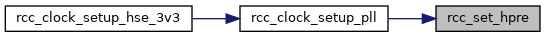## ◆ rcc_set_main_pll_hse()

 void rcc_set_main_pll_hse ( uint32_t pllm, uint32_t plln, uint32_t pllp, uint32_t pllq, uint32_t pllr )

Reconfigures the main PLL for a HSE source.

Any reserved bits are kept at their reset values.

Parameters
 pllm Divider for the main PLL input clock plln Main PLL multiplication factor for VCO pllp Main PLL divider for main system clock pllq Main PLL divider for USB OTG FS, SDMMC & RNG pllr Main PLL divider for DSI (for parts without DSI, provide 0 here)

Definition at line 680 of file rcc.c.

Referenced by rcc_clock_setup_pll().

Here is the caller graph for this function: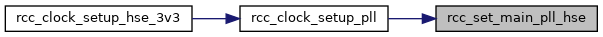## ◆ rcc_set_main_pll_hsi()

 void rcc_set_main_pll_hsi ( uint32_t pllm, uint32_t plln, uint32_t pllp, uint32_t pllq, uint32_t pllr )

Reconfigures the main PLL for a HSI source.

Any reserved bits are kept at their reset values.

Parameters
 pllm Divider for the main PLL input clock plln Main PLL multiplication factor for VCO pllp Main PLL divider for main system clock pllq Main PLL divider for USB OTG FS, SDMMC & RNG pllr Main PLL divider for DSI (for parts without DSI, provide 0 here)

Definition at line 656 of file rcc.c.

Referenced by rcc_clock_setup_pll().

Here is the caller graph for this function:## ◆ rcc_set_mco()

 void rcc_set_mco ( uint32_t mcosrc )

Select the source of Microcontroller Clock Output.

Exact sources available depend on your target. On devices with multiple MCO pins, this function controls MCO1

Parameters
 [in] mcosrc the unshifted source bits

Definition at line 207 of file rcc_common_all.c.

References RCC_CFGR, RCC_CFGR_MCO_MASK, and RCC_CFGR_MCO_SHIFT.

## ◆ rcc_set_pll_source()

 void rcc_set_pll_source ( uint32_t pllsrc )

Definition at line 602 of file rcc.c.

References RCC_PLLCFGR.

## ◆ rcc_set_ppre1()

 void rcc_set_ppre1 ( uint32_t ppre1 )

Definition at line 620 of file rcc.c.

References rcc_clock_scale::ppre1, and RCC_CFGR.

Referenced by rcc_clock_setup_pll().

Here is the caller graph for this function: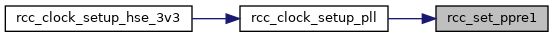## ◆ rcc_set_ppre2()

 void rcc_set_ppre2 ( uint32_t ppre2 )

Definition at line 611 of file rcc.c.

References rcc_clock_scale::ppre2, and RCC_CFGR.

Referenced by rcc_clock_setup_pll().

Here is the caller graph for this function: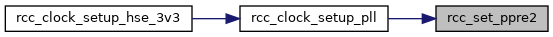## ◆ rcc_set_rtcpre()

 void rcc_set_rtcpre ( uint32_t rtcpre )

Definition at line 638 of file rcc.c.

References RCC_CFGR.

## ◆ rcc_set_sysclk_source()

 void rcc_set_sysclk_source ( uint32_t clk )

Definition at line 593 of file rcc.c.

References RCC_CFGR.

Referenced by rcc_clock_setup_pll().

Here is the caller graph for this function: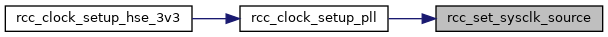## ◆ rcc_system_clock_source()

 uint32_t rcc_system_clock_source ( void )

Definition at line 695 of file rcc.c.

References RCC_CFGR.

## ◆ rcc_wait_for_osc_ready()

 void rcc_wait_for_osc_ready ( enum rcc_osc osc )

Wait for Oscillator Ready.

Block until the hardware indicates that the Oscillator is ready.

Parameters
 osc Oscillator ID

Definition at line 455 of file rcc.c.

References rcc_is_osc_ready().

Referenced by rcc_clock_setup_pll().

Here is the call graph for this function: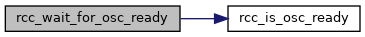Here is the caller graph for this function: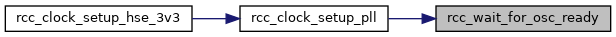## ◆ rcc_wait_for_sysclk_status()

 void rcc_wait_for_sysclk_status ( enum rcc_osc osc )

Definition at line 460 of file rcc.c.

Referenced by rcc_clock_setup_pll().

Here is the caller graph for this function: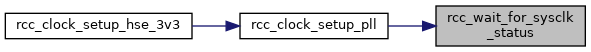## ◆ rcc_ahb_frequency

 uint32_t rcc_ahb_frequency
extern

Definition at line 48 of file rcc.c.

Referenced by rcc_clock_setup_pll().

## ◆ rcc_apb1_frequency

 uint32_t rcc_apb1_frequency
extern

Definition at line 49 of file rcc.c.

## ◆ rcc_apb2_frequency

 uint32_t rcc_apb2_frequency
extern

Definition at line 50 of file rcc.c.

## ◆ rcc_hse_12mhz_3v3

 const struct rcc_clock_scale rcc_hse_12mhz_3v3[RCC_CLOCK_3V3_END]
extern

Definition at line 50 of file rcc.c.

## ◆ rcc_hse_16mhz_3v3

 const struct rcc_clock_scale rcc_hse_16mhz_3v3[RCC_CLOCK_3V3_END]
extern

Definition at line 50 of file rcc.c.

## ◆ rcc_hse_25mhz_3v3

 const struct rcc_clock_scale rcc_hse_25mhz_3v3[RCC_CLOCK_3V3_END]
extern

Definition at line 50 of file rcc.c.

## ◆ rcc_hse_8mhz_3v3

 const struct rcc_clock_scale rcc_hse_8mhz_3v3[RCC_CLOCK_3V3_END]
extern

Definition at line 50 of file rcc.c.

## ◆ rcc_hsi_configs

 const struct rcc_clock_scale rcc_hsi_configs[RCC_CLOCK_3V3_END]
extern

Definition at line 50 of file rcc.c.